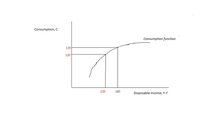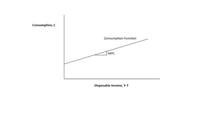# Marginal propensity to consume

﻿
Marginal propensity to consume

In economics, the marginal propensity to consume (MPC) is an empirical metric that quantifies induced consumption, the concept that the increase in personal consumer spending (consumption) occurs with an increase in disposable income (income after taxes and transfers). The proportion of the disposable income which individuals desire to spend on consumption is known as propensity to consume. Marginal propensity to consume (mpc) is the proportion of additional income that an individual desires to consume For example, if a household earns one extra dollar of disposable income, and the marginal propensity to consume is 0.65, then of that dollar, the household will spend 65 cents and save 35 cents.

Mathematically, the marginal propensity to consume (MPC) function is expressed as the derivative of the consumption (C) function with respect to disposable income (Y).$MPC=\frac{dC}{dY}$

OR$MPC = \frac{\Delta C}{\Delta Y}$, where ΔC is the change in consumption, and ΔY is the change in disposable income that produced the consumption.

Marginal propensity to consume can be found by dividing change in consumption by a change in income, or MPC=∆C/∆Y. The MPC can be explained with the simple example:

INCOME CONSUMPTION
120 120
180 170

Here ∆C= 50; ∆Y= 60 Therefore, MPC=∆C/∆Y= 50/60= 0.83 or 83%. For example, suppose you receive a bonus with your paycheck, and it's $500 on top of your normal annual earnings. You suddenly have$500 more in income than you did before. If you decide to spend $400 of this marginal increase in income on a new business suit, your marginal propensity to consume will be 0.8 ($400 / \$500).The above figure illustrates the consumption function. The slope of the consumption function tells us how much consumption increases when disposable income increases by one rupee. That is, the slope of the consumption function is the MPC.

The marginal propensity to consume is measured as the ratio of the change in consumption to the change in income, thus giving us a figure between 0 and 1. The MPC can be more than one if the subject borrowed money to finance expenditures higher than their income. One minus the MPC equals the marginal propensity to save (in a two sector closed economy), both of which are crucial to Keynesian economics and are key variables in determining the value of the multiplier.The MPC is the rate of change in the Average propensity to consume (APC ) . When income increases, the MPC falls but more than the APC. Contrariwise, when income falls, the MPC rises and the APC also rises but at a slower rate than the former. Such changes are only possible during cyclical fluctuations whereas in the short-run there is no change in the MPC and MPC<APC.

The MPC relies heavily upon the real (inflation-adjusted) rate of interest. A high rate of interest causes spending in the future to become increasingly attractive due to the intertemporal substitution effect on consumption. Because a rate increase primarily decreases the present value of lifetime wealth, the consumer relies on becoming a lender to offset this effect. In a two period model, as S(1+r) increases with the interest rate, so does future income[C= -(1+r)c +we(1+r)]. Therefore, every dollar of current income spent by the consumer is 1(1+r) dollars the consumer will not be able to spend in the second period.

Economists often distinguish between the marginal propensity to consume out of permanent income, and the marginal propensity to consume out of temporary income, because if consumers expect a change in income to be permanent, then they have a greater incentive to increase their consumption (Barro and Grilli, p. 417-8). This implies that the Keynesian multiplier should be larger in response to permanent changes in income than it is in response to temporary changes in income (though the earliest Keynesian analyses ignored these subtleties). However, the distinction between permanent and temporary changes in income is often subtle in practice, and it is often quite difficult to designate a particular change in income as being permanent or temporary. What is more, the marginal propensity to consume should also be affected by factors such as the prevailing interest rate and the general level of consumer surplus that can be derived from purchasing.MPC

## Contents

### MPC and the Multiplier:

MPC’s importance depends in the multiplier theory. The value of the multiplier-- is determined by MPC The higher the MPC, the higher the multiplier and vice-versa. The relationship between the multiplier and the propensity to consume is as follows:

                                                  Y=C+I
∆Y=∆C+∆I
Italic text                 ∆Y=c ∆Y+ ∆I                (where c is MPC)
∆Y- c ∆Y= ∆I
∆Y (1 -c) = ∆I
∆Y= ∆I/(1-c)
∆Y/∆I= 1/(1-c)
K= 1/(1-c)                    (where K is multiplier and K= ∆Y/∆I )


Since c is the MPC, the multiplier K is, by definition, equal to 1-1/c. The multiplier can also be derived from MPS (marginal propensity to save) and it is the reciprocal of MPS, K= 1/MPS

∆C/∆Y (MPC) ∆S/∆Y(MPS)[1-MPC] K (multiplier coefficient)
0 1 1
1/2 1/2 2
2/3 1/3 3
3/4 1/4 4
4/5 1/5 5
8/9 1/9 9
9/10 1/10 10
1 0 α

The above table shows that the size of the multiplier varies directly with the MPC and inversely with the MPS. Since the MPC is always greater than zero and less than one (i.e. 0<MPC <1), the multiplier is always between one and infinity (1<K<α). If the multiplier is one , it means that the whole increment of income is saved and nothing is spent because the MPC is zero. On the other hand, an infinite multiplier implies that MPC is equal one and the entire increment of income is spent on consumption. It will soon lead to full employment in the economy and then create a limitless inflationary spiral. But these are rare phenomenon. Therefore, the multiplier coefficient varies between one and infinity.

### Significance of MPC:

The MPC is the rate of change in the APC . When income increases, the MPC falls but more than the APC. Contrariwise, when income falls, the MPC rises and the APC also rises but at a slower rate than the former. Such changes are only possible during cyclical fluctuations whereas in the short-run there is no change in the MPC and MPC<APC. Keynes is concerned primarily with the MPC, for his analysis pertains to the short-run while the APC is useful in the long-run analysis. The post-Keynesian economists have come to the conclusion that over the long-run APC and MPC are equal and approximate 0.9. In the Keynesian analysis the MPC is given more prominence. Its value is assumed to be positive and less than unity which means that when income increases the whole of it is not spent on consumption. On the contrary, when income falls, consumption expenditure does not decline in the same proportion and never becomes zero. The Keynesian hypothesis is that the marginal propensity to consume is positive but less than unity (0<=∆C/∆Y <1) is of great analytical and practical significance. Besides telling us that consumption is an increasing function of income and it increases by less than the increment of income , this hypothesis helps in explaining 1) The theoretical possibility of general overproduction or ‘underemployment equilibrium’ and also 2) The relative stability of a highly developed industrial economy. For it imply that the gap between income and consumption at all high levels of income is too wide to be easily filled by investment with the possible consequences that the economy may fluctuate around underemployment equilibrium. Thus the economic significance of the MPC lies in filling the gap between income and consumption through planned investment to maintain the desired level of income.

#### MPC and nature of country:

The MPC is higher in the case of poor than in case of rich people. The greater is any man’s income, the more of his basic human needs will have already been met, and the greater will be the tendency for him to save in order to provide for future. The marginal propensity to save of the richer classes shall be greater than that of the poorer classes. If, at any time, it is desired to increase aggregate consumption, then the purchasing power should be transferred from the richer classes (with low propensity to consume) to the poorer classes (with a higher propensity to consume). Likewise, if it is desired to reduce community consumption, the purchasing power must be taken away from the poorer classes by taxing consumption. The marginal propensity to consume is higher in a poor country and lower in the case of rich country. The reason is same as stated above. In the case of rich country, most of the basic wants of the people have already been satisfied, and all the additional increments of income are saved, resulting in a higher marginal propensity to save but in a lower marginal propensity to consume. In a poor country, on the other hand, most of the basic wants of the people remain unsatisfied so that additional increments of income go to increase consumption, resulting in a higher marginal propensity to consume but in a lower marginal propensity to save.it is on account of this that the MPC is higher in the backward and underdeveloped countries of Asia and Africa,a nd lower in the advanced and developed countries like the USA, the UK, West Germany etc.